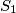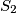### IMO Shortlist 1987 problem 10

Kvaliteta:
Avg: 0,0
Težina:
Avg: 0,0
Let$S_1$ and$S_2$ be two spheres with distinct radii that touch externally. The spheres lie inside a cone$C$, and each sphere touches the cone in a full circle. Inside the cone there are$n$ additional solid spheres arranged in a ring in such a way that each solid sphere touches the cone$C$, both of the spheres$S_1$ and$S_2$ externally, as well as the two neighboring solid spheres. What are the possible values of$n$?

Proposed by Iceland.
Izvor: Međunarodna matematička olimpijada, shortlist 1987